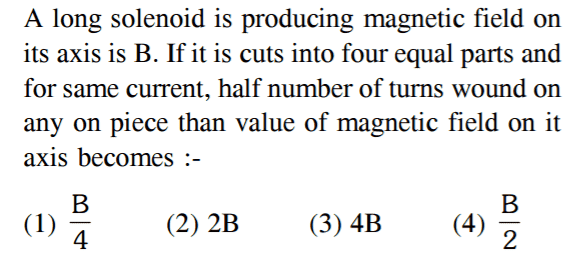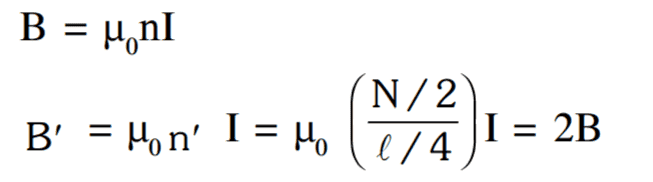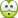# Magnetic field due to a solenoid

## Homework Statement## The Attempt at a Solution

I am having trouble interpreting the language of the problem statement . What does it mean that "half number of turns are wound " ?

When the solenoid is cut , the length becomes 1/4th of the original and number of turns also become 1/4th of the original .

I think the problem is not difficult if only I could understand the question .

Thanks .

#### Attachments

•Merlin3189

mfb
Mentor
That is a strange question. Are the four equal parts picked up again later?
If not, I don't understand that part. I would assume the density of the windings (windings per length) is halved.

•Jahnavi
Are the four equal parts picked up again later?

I think so .

I would assume the density of the windings (windings per length) is halved.

How ?

What does it mean "half number of turns wound on any one piece " ?

Last edited:
mfb
Mentor
Well, the sentence does not use correct English grammar, so it is a bit speculation, but I interpreted it as "For each quarter, we halve the winding density". For the following question this means the quarters don't matter.
I think so .
Can you show the full problem with its context?

Can you show the full problem with its context?

That is all I have . This is an objective type MCQ from a test paper .

I interpreted it as "For each quarter, we halve the winding density".

This interpretation gives option 4) . Right ?

For the following question this means the quarters don't matter.

Could you explain this .

mfb
Mentor
This interpretation gives option 4) . Right ?
Sure.
Could you explain this .
You didn't use the part about quarters to arrive at the answer of B/2, right?

•JahnaviI think what they ( paper setters ) are trying to do is halving the total number of turns (before the solenoid is cut ) and then fitting those N/2 turns in the length L/4 ( after the solenoid is cut in four ) . Strange interpretation.

Please let me know if the official solution makes sense to you .

#### Attachments

mfb
Mentor
I can see how you could interpret the problem that way, but the phrasing is unnecessarily complicated and ambiguous.

•Jahnavi
Thanks !